# Q Solutions Prem Sattsangi Copyright 2008 1 HYDROGENBONDS5

• Slides: 14
Download presentation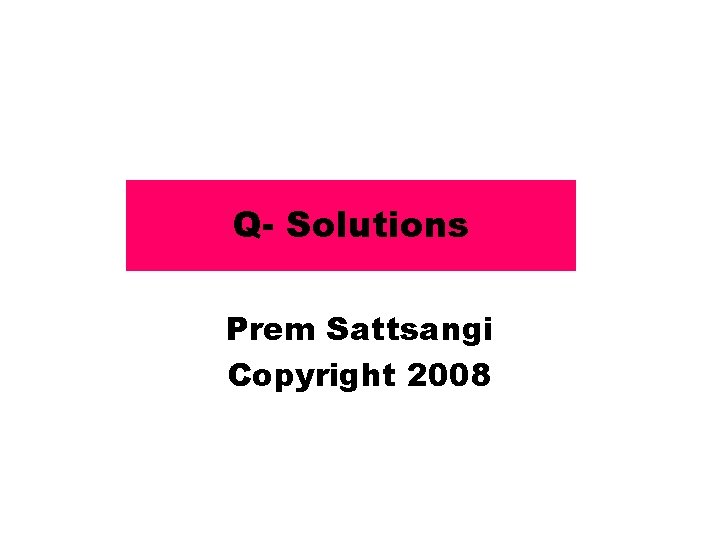Q- Solutions Prem Sattsangi Copyright 2008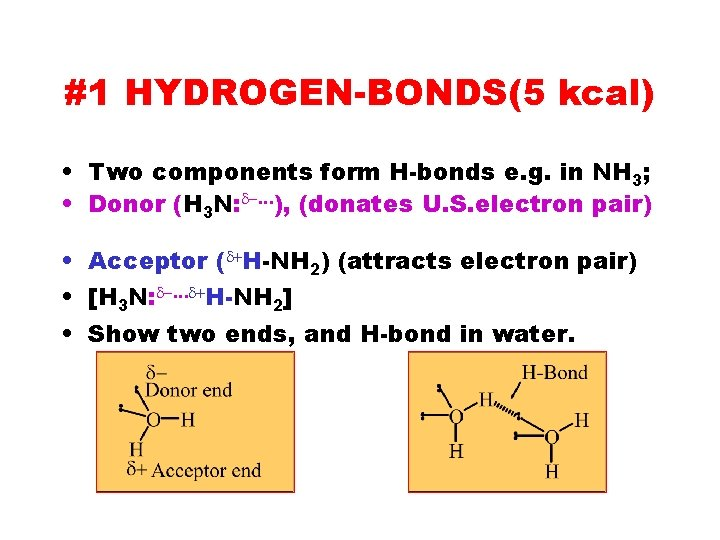#1 HYDROGEN-BONDS(5 kcal) • Two components form H-bonds e. g. in NH 3; • Donor (H 3 N: d-…), (donates U. S. electron pair) • Acceptor (d+H-NH 2) (attracts electron pair) • [H 3 N: d-…d+H-NH 2] • Show two ends, and H-bond in water.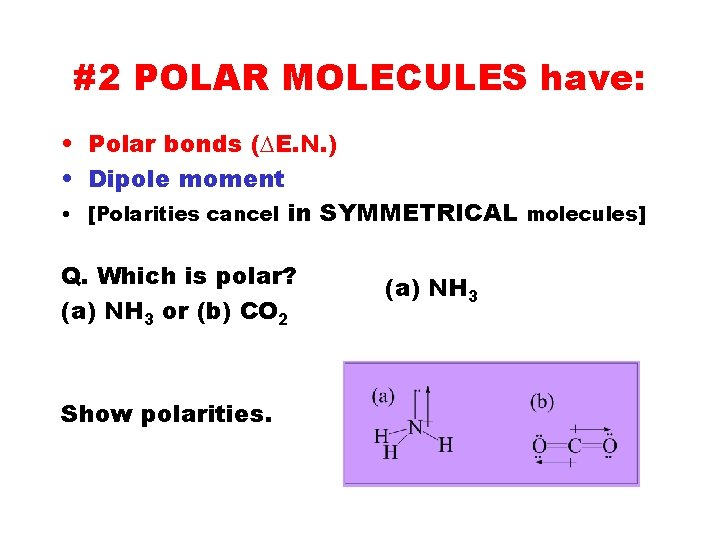#2 POLAR MOLECULES have: • Polar bonds (DE. N. ) • Dipole moment • [Polarities cancel in SYMMETRICAL molecules] Q. Which is polar? (a) NH 3 or (b) CO 2 Show polarities. (a) NH 3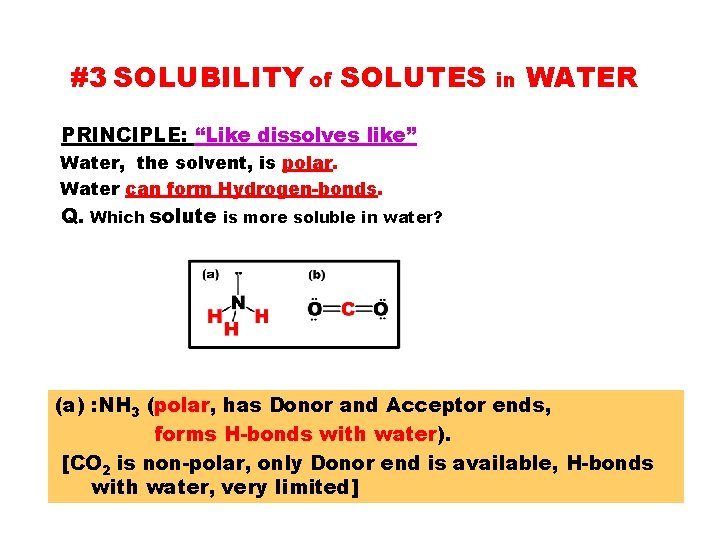#3 SOLUBILITY of SOLUTES in WATER PRINCIPLE: “Like dissolves like” Water, the solvent, is polar. Water can form Hydrogen-bonds. Q. Which solute is more soluble in water? (a) : NH 3 (polar, has Donor and Acceptor ends, forms H-bonds with water). [CO 2 is non-polar, only Donor end is available, H-bonds with water, very limited]#4 Table 4. 1: Solubility Guidelines for Common Ionic Compounds in Water • • SOLUBLE: Acids Gr. IA, and NH 4+ salts Acetates, and Nitrates • • • Halides (Except: Ag+, Pb 2+, Hg 22+) Sulfates (Except: Pb 2+, Hg 22+) Ba 2+, Sr 2+ ) Ag+ Borderline ? • INSOLUBLE: • Except: • Carbonates • Phosphates Gr. IA, NH 4+ • Hydroxides • Except: Gr. IA, NH 4+ Ca 2+, Sr 2+, Ba 2+ Mg(OH)2(Insoluble)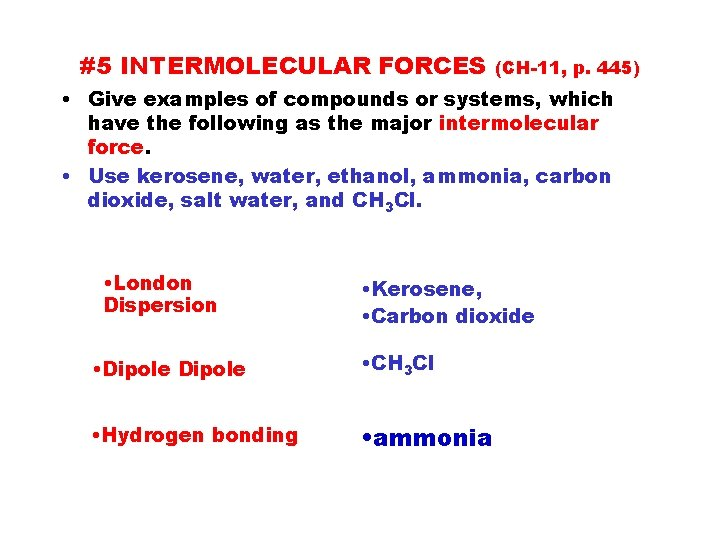#5 INTERMOLECULAR FORCES (CH-11, p. 445) • Give examples of compounds or systems, which have the following as the major intermolecular force. • Use kerosene, water, ethanol, ammonia, carbon dioxide, salt water, and CH 3 Cl. • London Dispersion • Kerosene, • Carbon dioxide • Dipole • CH 3 Cl • Hydrogen bonding • ammonia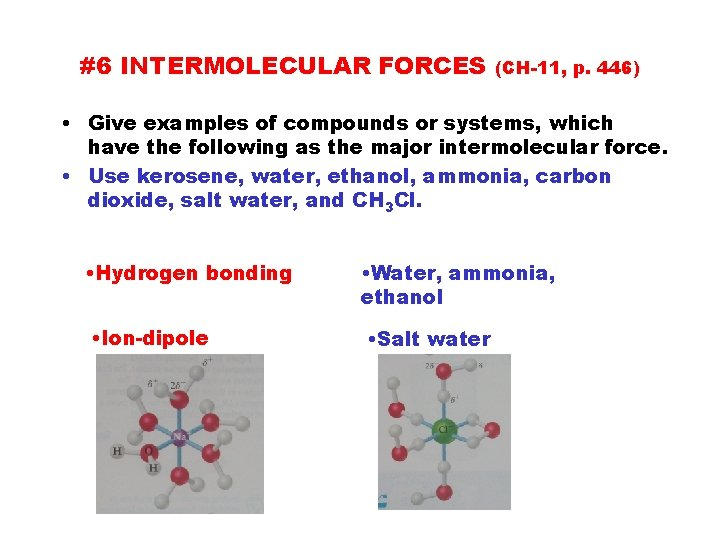#6 INTERMOLECULAR FORCES (CH-11, p. 446) • Give examples of compounds or systems, which have the following as the major intermolecular force. • Use kerosene, water, ethanol, ammonia, carbon dioxide, salt water, and CH 3 Cl. • Hydrogen bonding • Water, ammonia, ethanol • Ion-dipole • Salt water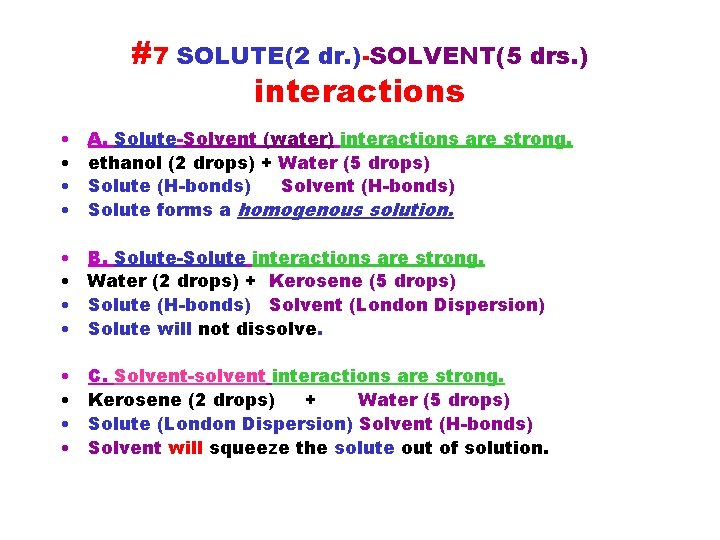#7 SOLUTE(2 dr. )-SOLVENT(5 drs. ) interactions • • A. Solute-Solvent (water) interactions are strong. ethanol (2 drops) + Water (5 drops) Solute (H-bonds) Solvent (H-bonds) Solute forms a homogenous solution. • • B. Solute-Solute interactions are strong. Water (2 drops) + Kerosene (5 drops) Solute (H-bonds) Solvent (London Dispersion) Solute will not dissolve. • • C. Solvent-solvent interactions are strong. Kerosene (2 drops) + Water (5 drops) Solute (London Dispersion) Solvent (H-bonds) Solvent will squeeze the solute out of solution.#8 Solute/Solvent Interactions • For a mixture of Water (2 drops) + Kerosene (5 drops) True or False: • ___ Solute/Solute interactions are strong. • ___ Solute/Solvent interactions are strong. • ___ Solvent/Solvent interactions are strong. • ___ Solute is squeezed out of solution. • ___ Solute comes out of solution.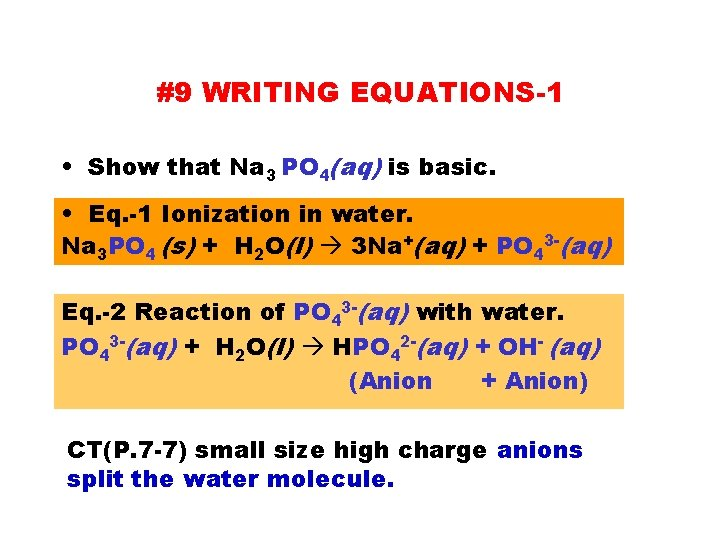#9 WRITING EQUATIONS-1 • Show that Na 3 PO 4(aq) is basic. • Eq. -1 Ionization in water. Na 3 PO 4 (s) + H 2 O(l) 3 Na+(aq) + PO 43 -(aq) Eq. -2 Reaction of PO 43 -(aq) with water. PO 43 -(aq) + H 2 O(l) HPO 42 -(aq) + OH- (aq) (Anion + Anion) CT(P. 7 -7) small size high charge anions split the water molecule.#10 WRITING EQUATIONS-2 • Show that Al. Cl 3(aq) is acidic. Eq. -1 Ionization in water. Al. Cl 3 (aq) + H 2 O(l) Al 3+(aq) + 3 Cl-(aq) Eq. -2 Reaction with water. Al 3+(aq) + H 2 O(aq) Al. OH 2+(aq) + H+(aq) (Cation + Cation) CT(P. 7 -6) small size high charge cations split the water molecule.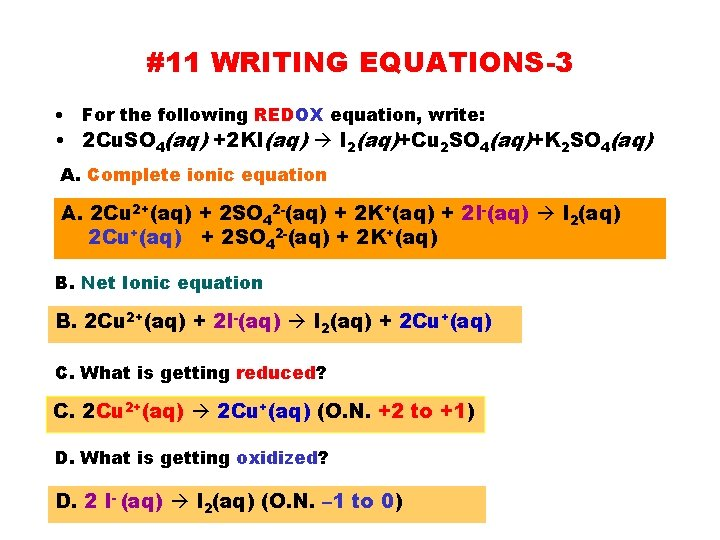#11 WRITING EQUATIONS-3 • For the following REDOX equation, write: • 2 Cu. SO 4(aq) +2 KI(aq) I 2(aq)+Cu 2 SO 4(aq)+K 2 SO 4(aq) A. Complete ionic equation A. 2 Cu 2+(aq) + 2 SO 42 -(aq) + 2 K+(aq) + 2 I-(aq) I 2(aq) 2 Cu+(aq) + 2 SO 42 -(aq) + 2 K+(aq) B. Net Ionic equation B. 2 Cu 2+(aq) + 2 I-(aq) I 2(aq) + 2 Cu+(aq) C. What is getting reduced? C. 2 Cu 2+(aq) 2 Cu+(aq) (O. N. +2 to +1) D. What is getting oxidized? D. 2 I- (aq) I 2(aq) (O. N. – 1 to 0)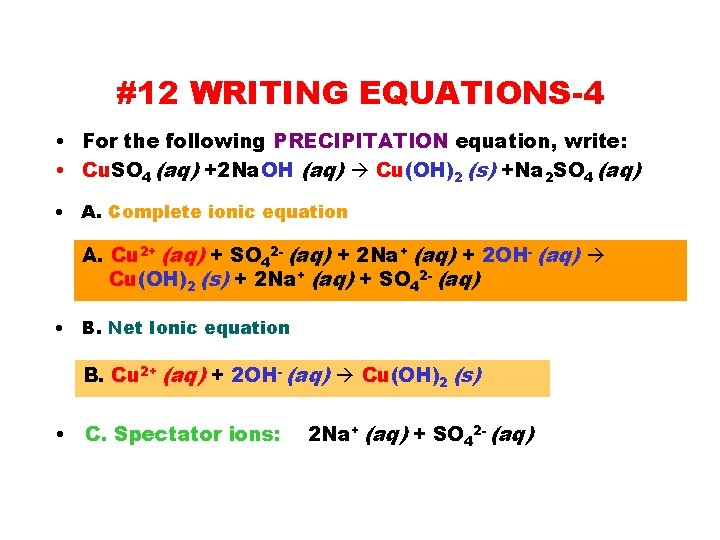#12 WRITING EQUATIONS-4 • For the following PRECIPITATION equation, write: • Cu. SO 4 (aq) +2 Na. OH (aq) Cu(OH)2 (s) +Na 2 SO 4 (aq) • A. Complete ionic equation A. Cu 2+ (aq) + SO 42 - (aq) + 2 Na+ (aq) + 2 OH- (aq) Cu(OH)2 (s) + 2 Na+ (aq) + SO 42 - (aq) • B. Net Ionic equation B. Cu 2+ (aq) + 2 OH- (aq) Cu(OH)2 (s) • C. Spectator ions: 2 Na+ (aq) + SO 42 - (aq)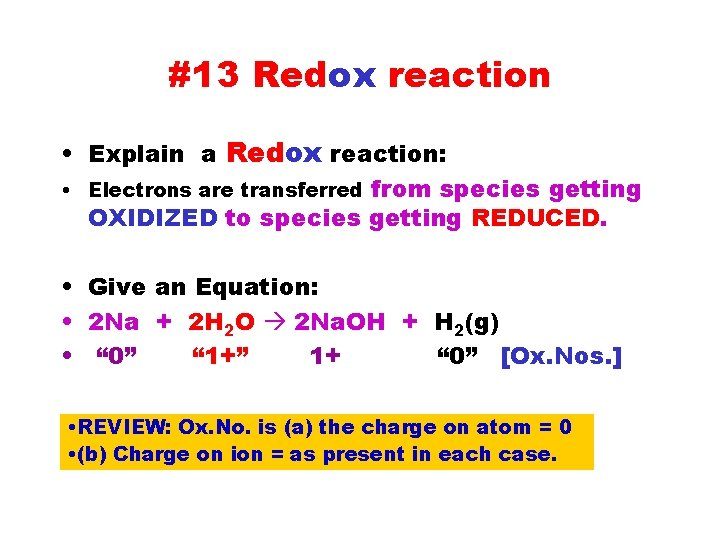#13 Redox reaction • Explain a Redox reaction: • Electrons are transferred from species getting OXIDIZED to species getting REDUCED. • Give an Equation: • 2 Na + 2 H 2 O 2 Na. OH + H 2(g) • “ 0” “ 1+” 1+ “ 0” [Ox. Nos. ] • REVIEW: Ox. No. is (a) the charge on atom = 0 • (b) Charge on ion = as present in each case.